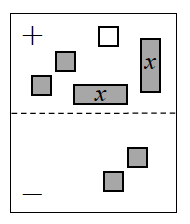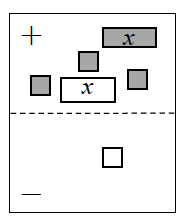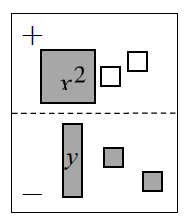### Home > AC > Chapter 2 > Lesson 2.1.6 > Problem2-59

2-59.

Find a simplified algebraic expression for each Expression Mat below.1.2-59a HW eToolDetermine the zero pairs and remove them. What is an expression for the remaining tiles?

$2x−1$

1.On this mat, both $x$-tiles in the (+) region can be removed, since they make a zero pair. Next, flip the $-1$ unit tile in the ($-$) region to the ($+$) region, and then simplify.

1.Unlike in parts (a) and (b), this problem has no zero pairs.
However, here is an un-simplified expression to get you started.

$x^2+(−1)+(−1)-(y+1+1)$
Can you simplify that expression?

Use the eTool below to find the simplified algebraic expression.
Click the link at right for the full version of the eTool: 2-59a HW eToolUse the eTool below to find the simplified algebraic expression.
Click the link at right for the full version of the eTool: 2-59b HW eToolUse the eTool below to find the simplified algebraic expression.
Click the link at right for the full version of the eTool: 2-59c HW eTool